## Table of ContentOpen Access

ARTICLE

# A Consistent Time Level Implementation Preserving Second-Order Time Accuracy via a Framework of Unified Time Integrators in the Discrete Element Approach

Tao Xue1, Yazhou Wang2, Masao Shimada2, David Tae2, Kumar Tamma2,*, Xiaobing Zhang1,*

1 School of Energy and Power Engineering, Nanjing University of Science and Technology, Nanjing, 210094, China
2 Department of Mechanical Engineering, University of Minnesota-Twin Cities, Minneapolis, 55455, USA

* Corresponding Authors: Kumar Tamma. Email:; Xiaobing Zhang. Email:Computer Modeling in Engineering & Sciences 2023, 134(3), 1469-1487. https://doi.org/10.32604/cmes.2022.021616

## Abstract

In this work, a consistent and physically accurate implementation of the general framework of unified second-order time accurate integrators via the well-known GSSSS framework in the Discrete Element Method is presented. The improved tangential displacement evaluation in the present implementation of the discrete element method has been derived and implemented to preserve the consistency of the correct time level evaluation during the time integration process in calculating the algorithmic tangential displacement. Several numerical examples have been used to validate the proposed tangential displacement evaluation; this is in contrast to past practices which only seem to attain the first-order time accuracy due to inconsistent time level implementation with different algorithms for normal and tangential directions. The comparisons with the existing implementation and the superiority of the proposed implementation are given in terms of the convergence rate with improved numerical accuracy in time. Moreover, several schemes via the unified second-order time integrators within the framework of the GSSSS family have been carried out based on the proposed correct implementation. All the numerical results demonstrate that using the existing state-of-the-art implementation reduces the time accuracy to be first-order accurate in time, while the proposed implementation preserves the correct time accuracy to yield second-order.

## Keywords

1  Introduction

In order to numerically describe the behavior of granular materials (not continuous body) represented as a collection of large number of distinct particles, the discrete/distinct element method (DEM), proposed in , is being widely used for its ease of implementation and versatility. In the DEM technique, each particle element can undergo large displacement and rotation, and the deformations of individual particle elements are assumed to be small, compared with their displacements; hence we can treat the elements as rigid bodies and the deformation is usually described in terms of the relative normal displacement and the relative tangential displacement between the particles in contact. Consequently, the evaluation of those two displacements has a significant influence on both the dynamic response of particles and the numerical accuracy of the simulation.

One of the common applications for the DEM is particle packing. Various packing methods using DEM have been studied previously such as, Formation of a pile, i.e., sand piling process , pouring or depositing particles under gravity . The DEM particle packing is also used in pharmaceutical field for powder tableting [13,14]. Another field of application using DEM is simulating particle flow; and a commonly studied example is ball mill mixer simulation; some of which include, horizontally rotating drums , tumbling ball mill . Drum and mill are commonly used in industries for mixing, drying or coating subjects; thus, there is extensive interest among researchers in this study. Besides, the DEM is still studied and utilized in recent studies such as solid-liquid flow simulation , impact disruption of cohesive soft sphere simulation , granular shear flows of dry flexible fibers  and non-spherical complex granular simulation .

Furthermore, the DEM not only simulates granular flow, but it can also be used for solid body analysis and assessing fracture as studied in . Recently, the DEM has been expanded to be able to solve multi-physics applications where granular particles interact with a continuous body, be it structural or thermodynamics. An Extended Discrete Element Method (XDEM) has been proposed in  which couples continuous body analysis method and DEM together without losing small scale information from commonly used volume averaging concept. The XDEM allows those microscale information from particles to accurately influence a macroscopic body. The XDEM opens up a wide variety of possible physical applications in many fields of study.

In transient dynamic simulation of the DEM type problems, contact forces and particle motion need to be evaluated and updated at every time step. The widely used time integrations in the DEM numerical simulations include the explicit Euler method, fourth-order Runge-Kutta (RK-4) method, and central difference method/velocity verlet method, etc. It is straightforward to implement these methods in the DEM simulation; however, these explicit time stepping techniques possess several numerical disadvantages in addition to the conditionally stable features; for example, the Euler methods are first-order accurate in time, RK-4 method requires additional computations of high-order derivatives of differential variables [28,29], and the central difference method is a non-dissipative scheme, i.e., it cannot introduce numerical dissipation if one needs to. Also, past practices poorly model the tangential physics of particle interactions. Recently, a unified time integration framework, under the umbrella of the so-called generalized single-step single-solve (GSSSS) family of algorithms, including not only implicit, but also explicit single-step single-solve algorithms, which can also be written in a linear three-step form, has been proposed in . This single simulation tool kit, which has been originally developed in the field of computational structural dynamics, encompasses not only most existing algorithms that are second-order time accurate and are commonly used over the past 50 years or so, but it additionally inherits a wide variety of new implicit and explicit time integration schemes also with second-order time accuracy, including the explicit central difference method as a special case. To implement these time integrations in DEM and preserve the numerical accuracy of the algorithms, the contact force is required to be evaluated in a consistent way with the selected time integrations in both normal and tangential directions. According to our knowledge, most of the existing literature have been embedding the first-order accurate updating evaluation of tangential displacement into the first/second-order accurate time integrations. The specifics are shown in Section 2, leading to reduced accuracy.

In this work, the main objective is to show the consistent implementation of the GSSSS family of algorithms to the simulation of granular assemblies with DEM while retaining all the advantages of numerous methods within the present framework of algorithms. It is worthy to mention that one major drawback of the DEM technique, as well as the other particle based methods or meshless methods , is contact detection or neighboring particle search. To circumvent this issue, the linked list is employed to detect the contacting neighbor particles. In addition, Open Multi-Processor (Open MP) is implemented to reduce the simulation computational time. The outline of the rest of the paper is as follows. Section 2 presents the basic theory of DEM formulations and both the linear and the nonlinear model of contact forces and torques are presented in detail. The GSSSS unified time integrators are highlighted in Subsection 2.2 and the specifics of the proposed consistent tangential displacement evaluation is derived and compared with the existing tangential displacement evaluation technique carefully. The effectiveness of the proposed consistent tangential displacement evaluation is demonstrated and illustrated using simple numerical examples in Section 3. Finally, the conclusions are drawn and presented in Section 4. This study shows that the DEM with explicit schemes within the GSSSS family of algorithms achieves and consistently preserves second-order time accuracy; the consistent time level implementation and preserving the second-order time accuracy for the presented results for a wide variety of time integrators in the present unified framework include features such as no overshoot in displacement or no overshoot in velocity or no overshoot in both displacement and velocity for a given set of initial conditions of the particles and differs from algorithm to algorithm.

2  Discrete Element Method with the Explicit GSSSS Family of Algorithms

2.1 Basic DEM Formulations

DEM is a numerical simulation technique for a system of small “distinct” particles/elements, such as powders and granular materials. For the sake of simplicity, we assume the shape of every particle/element in a system is a disk in the two-dimensional Euclidean space E2 or a sphere in the three dimensional Euclidean space E3, although DEM can treat any shapes of elements in general, see . In DEM, we assume that each particle is rigid, i.e., no deformation; however, particles are allowed to overlap slightly one another at the contact points.

Let qi(t):[0,)Rndim denote the position component vector of the ith particle (i=1,2,,nele) with respect to the Cartesian coordinate frame B, where nele and ndim denote the number of particles/elements in the system and number of dimension, respectively. Suppose that the ith and jth particles are in contact at time t as shown in Fig. 1a. The overlap (penetration) between particle i and j, which is denoted by nδijR, is defined as

nδij=(ri+rj)dij(1)

where ri and rj denote the radii of the ith and jth particles, respectively; and dij is the center distance between the particles i and j and dij:=qjqi=(qjqi)(qjqi) is the center distance between the particles i and j. We assume the overlap is sufficiently small relatively to the particle sizes. If the inequality dij<ri+rj is met, the overlap nδij>0 occurs; and the contact forces between patricles i and j are taken into consideration. Define the unit normal vector between these particles as nij:=qjqiqjqi=qjqidij,nij=1. The translational velocity component vectors of particles i and j (at the center points CiEndim and CjEndim, respectively) with respect to B are given as the (total) time derivatives of the position components vectors, i.e., vi=q˙i and vj:=q˙j, respectively (˙d/dt denotes the total time derivative of ). Let ωi and αi denote the angular velocity and angular acceleration vectors of particle i, respectively (for i=1,2,,nele). Therefore, the velocity at point PiEndim, as shown in Fig. 1a, vPi, can be written as vPi=vi+ωi×ri=vi+riωi×nij, where ri=rinij. Similarly, vPj, the velocity of point Pj, can be written as vPj=vj+ωj×rj=vjrjωj×nij, where rj=rjnij. Hence, the relative velocity vector at point Pi with respect to point Pj is obtained as

vPi/Pj=vPivPj=vi/j+(riωi+rjωj)×nij(2)

where vi/j:=vivj denotes the relative translational velocities of particle i with respect to j. Since the projection of vPi/Pj parallel to nij is given as

nvPi/Pj=(vPi/Pjnij)nij=(vi/jnij)nij,(3)

the projection of vPi/Pj perpendicular to nij, which is obtained by subtracting nvPi/Pj from vPi/Pj, can be written as

svPi/Pj=vPi/Pj(vPi/Pjnij)nij(4)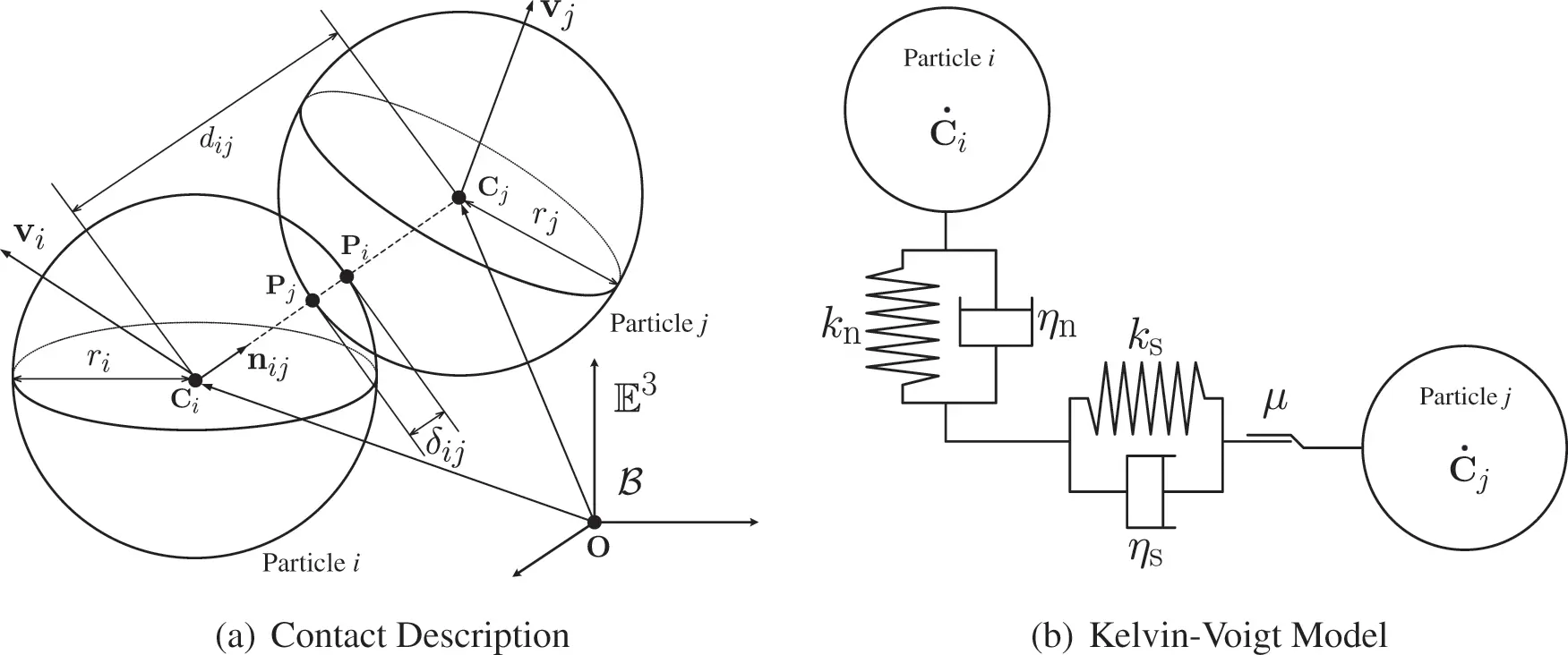Figure 1: (a) Pictorial illustration of particles i and j in contact; and (b) the Kelvin-Voigt model for particles i and j in contact. OE3 is an origin of a cartesian coordinate frame B for E3 with a right-handed orthonormal basis for the associated vector space

Note that nvPi/Pj and svPi/Pj are the relative velocity vector of point Pi with respect to point Pj in the normal (nij) and tangential (tij) directions, respectively. Substituting Eqs. (2) into (4) yields:

svPi/Pj=vi/j(vi/jnij)nij+(riωi+rjωj)×nij (5)

Hence, tij=svPi/Pj||svPi/Pj||,tij=1.

Similarly, we can derive the relative acceleration vectors of point Pi with respect to point Pj in the normal (nij) and tangential (tij) directions. Since the accelerations at Pi and Pj are given as aPi=ai+ωi×(ωi×ri)+αi×ri=ai+riωi×(ωi×nij)+riαi×nij and aPj=aj+ωj×(ωj×rj)+αj×rj=ajrjωj×(ωj×nij)rjαj×nij, where aiRndim and ajRndim are the linear acceleration vectors of particles i and j, respectively, the relative acceleration vector at point Pi with respect to point Pj may be obtained as

aPi/Pj=aPiaPj=ai/j+(riαi+rjαj)×nij+ri[(ωiωi)(ωiωi)Indim]nij+rj[(ωjωj)(ωjωj)Indim]nij(6)

where ai/j:=aiaj denotes the relative translational accelerations of particle i with respect to j, and IndimRndim×ndim denotes the identity tensor. Hence, the relative acceleration vector of point Pi with respect to point Pj in the normal (nij) and tangential (tij) directions are obtained as

naPi/Pj=(aPi/Pjnij)nijandsaPi/Pj=(aPi/Pjtij)tij(7)

respectively.

Contact Forces and Torques

The resultant contact force and torque acting on particle i are defined as the summations of the contact forces and torques, respectively, acting on particle i from all particles j(i) that are in contact with particle i. The contact forces due to particle(s) j can be modeled as follows.

Linear Model: The contact force acting on particle i from particle j can be uniquely decomposed into the contact forces in the normal direction (nij) and tangential direction (tij) as fij=nfij+sfijRndim. In DEM, the contact forces are usually modeled by the Kelvin-Voigt model, as shown in Fig. 1b; hence, both the normal and tangential contact forces on particle i due to particle j have the stiffness/spring and damping terms, and the friction on the surface is also considered. For the normal contact force, nfij, using the stiffness and damping constants in the normal direction, kn and ηn, respectively, we have

nfij=kn(nδij)+ηn(nvPi/Pj)=[kn(nδij)+ηn(vi/jnij)]nij(8)

where nδij is the relative displacement vector between particle i and j in the normal direction, i.e., nδijRndim, may be defined as the displacement while the particles i and j are in contact for the time interval, [tc1,tc2][0.), i.e.,

nδij=tc1tc2nvPi/Pjdt=nδijnij=[(ri+rj)dij]nij(9)

The viscous damping coefficient ηn in Eq. (8) is usually selected as ηn=2lnCRm^ijknπ2+(lnCR)2, where CR[0,1]R+ denotes the coefficient of restitution, and m^ij, which is sometimes called the reduced mass, is defined as

m^ij:=[mi1+mj1]1=mimjmi+mj(10)

Similarly, the tangential contact force, sfij, can be written as

sfij=ks(sδij)+ηs(svPi/Pj)(11)

where ks and ηs are the stiffness and damping coefficients in the tangential direction, respectively. The tangential relative velocity, svPi/Pj, in the equation above is given by Eq. (5). The relative displacement vector between particles i and j in the tangential direction, i.e., sδijRndim, may be defined as the displacement while the particles i and j are in contact for the time interval, [tc1,tc2][0.), i.e.,

sδij=tc1tc2svPi/Pjdt(12)

We usually take ηsηn unless otherwise the values of the damping coefficients are critical in the simulation. If the condition ||sfij||>μ||nfij||, where μ>0 denotes the coefficient of friction, is met, the “slip” between particles i and j on the surface at the contact point occurs, and therefore, the tangential contact force, given in Eq. (11), is replaced with the frictional force, sfij=μ||nfij||tij with nfij, given in Eq. (8). Once we get all the contact forces, fij, acting on particle i, we can finally obtain the resultant contact force on particle i as fi=jincontifij, where nconti denotes the number of the particles that are in contact with particle i at time t. On the other hand, the resultant torque on particle i can be written in the form hi=jincontiri×sfij.

Nonlinear Model: There exist several nonlinear models developed for DEM in the literature to obtain more accurate, realistic simulation results. A common nonlinear model of DEM, proposed in , is based on the classical Hertzian contact theory and Mindlin theory [38,39]. The normal contact force, nfij, based on the Hertizian contact theory for two spheres, is still defined in the same form as Eq. (8),

nfij=kn(nδij)ηn(nvPi/Pj)(13)

However, as opposed to the linear model, nδij and stiffness kn are defined as

nδij=(nδij3/2)nij(14)

kn=43r^ijE^ij(15)

with nδij, defined as in Eq. (1), r^ij:=[ri1+rj1]1=rirjri+rj, and E^ij:=[1νi2Ei+1νj2Ej]1=EiEj(1νi2)Ej+(1νj2)Ei where Ei and νi are the Young modulus and Poisson ratio of particle i, respectively. For two spherical particles with the same radius (r=ri=rj) and the same material properties (E=Ei=Ej and ν=νi=νj), the stiffness defined in Eq. (15) may be written in the form, kn=E2r3(1ν2). In the case of a spherical particle i with a wall, we assume rj+, i.e., r^ri; and thereby, kn=4ri3EiEwall(1νi2)Ewall+(1νwall2)Ei, where Ewall and νwall are the Young modulus and Poisson ratio of the wall. According to , the normal damping coefficient ηn in Eq. (13) is suggested to be ηn=κm^ijkn(nδij1/4), in which m^ij is as defined in Eq. (10). For κ>0, which can be regarded as dependent on the coefficient of restitution between two sphere particles or between a sphere particle and a wall, it is to be determined empirically by analysts (Usually, α[0.01,1]). Note that the nvPi/Pj in Eq. (13) is still defined as given in Eq. (3).

On the other hand, the tangential contact force, sfij, for a nonlinear model is defined, based on the Hertzian contact theory and Mindlin theory [38,39] with the assumption that “no-slip” is allowed between two sphere particles i and j, as

sfij=ks(sδij)ηs(svPi/Pj)(16)

with

ks=[18bij2νiGi+2νjGj]1,bij:=r^ijnδij(17)

where Gi:=Ei/[2(1+νi)] is called the shear modulus of particle i, and bij is the radius of a contact area between sphere particles i and j. For two sphere particles with the same radius (r=ri=rj) and the same material properties (E=Ei=Ej and ν=νi=νj, i.e., G:=Gi=Gj), we have ks=2G2ν2r(nδij). In the case of the contact between sphere particle i and a wall, we assume the wall (rj+) is rigid, i.e., Ej+, since the elastic tangential displacement of the wall due to the contact with particle i is usually negligible; hence, Eq. (17) leads to the following expression: ks=8Gi2νiri(nδij). For most applications, we take the same value for the normal and tangential damping coefficients: ηsηn.

2.2 Transient Analysis: A Consistent Time Level Implementation of Unified Time Integrators

Applying the Newton-Euler equation to particle i for i=1,2,,nele, we get

Miai =fiappl(18)

Jiαi=hiappl(19)

where Mi=miIndimRndim×ndim, with the identity tensor IndimRndim×ndim, and aiRndim denote the mass tensor and the acceleration vector of particle i, respectively; and JiRndim×ndim and αiRndim denote the inertia tensor (with respect to the center of mass) and the angular acceleration vector of particle i, respectively. The right-hand side of Eq. (18), i.e., fiapplRndim, is the applied force on particle i, and it includes any force acting on the particle, including conservative and nonconservative forces, as well as the contact forces described in the previous subsection. Likewise, hiapplRndim in Eq. (19) is the applied torque vector of particle i.

In a unified format for all particles (i=1,2,,nele) in the system, Eqs. (18) and (19) can be written in the form Ma=fappl and Jα=happl, respectively, where M=diag(M1,M2,,Mnele)RN×N, J=diag(J1,J2,,Jnele)RN×N, a=(a1T,a2T,,aneleT)TRN, α=(α1T,α2T,,αneleT)TRN, fappl=(f1applT,f2applT,,fneleapplT)TRN, h=(h1applT,h2applT,,hneleapplT)TRN with N:=ndimnele. Or simply,

[M00J]M(aα)a=(fapplh)ft[0,)(20)

Time Discretization

Consider the temporal discretization of the governing equations for a time interval I=[t0,tf][0,) split into subintervals, i.e., I=[t0,tf]=n=0f1[tn,tn+1], in which t0 and tf denote the initial and final time levels of simulation, respectively. The time step size is defined as Δt:=tn+1tn>0, and it is assumed to be constant for simplicity. Temporally discretizing Eq. (20) by way of the normalized time weighted residual methodology  results in the following algorithmic framework:

Ma~n+1=f(q~n+1,v~n+1,tn+W1)(21)

where the algorithmic acceleration, velocity, and configuration vectors are defined as

a~n+1=an+W1Λ6Δan+1v~n+1=vn+ΔtW1an,q~n+1=qn+ΔtW1vn+Δt22W2an(22)

respectively, for n=0,1,,f1. The associated update equations to advance from t=tn to t=tn+1 are

qn+1=qn+Δtvn+Δt22an+λ3Δan+1Δt2vn+1=vn+Δtan+Δtλ5Δan+1,an+1=an+Δan+1(23)

The algorithmic parameters, also known as the DNA [discrete numerically assigned] markers, are defined with the input scalar algorithmic parameters (ρmin,ρmax,ρs), denoting the minimum principal root, the maximum principal root, and the spurious root , respectively, together with η, as

W1=W2=11+ρs,W1Λ6=2+ρmin+ρmax+ρsρminρmaxρs(1+ρmin)(1+ρmax)(1+ρs)λ3=η(1+ρmin)(1+ρmax),λ5=3+ρmin+ρmaxρminρmax2(1+ρmin)(1+ρmax)(24)

for the U0(ρmin,ρmax,ρs) family, and

W1=3+ρmin+ρmaxρminρmax2(1+ρmin)(1+ρmax),W2=2(1+ρmin)(1+ρmax)W1Λ6=2+ρmin+ρmax+ρsρminρmaxρs(1+ρmin)(1+ρmax)(1+ρs),λ3=η2(1+ρs),λ5=11+ρs(25)

for the V0(ρmin,ρmax,ρs) family. The U0 and V0 represent zero-order overshooting behaviors in the configuration and the velocity fields, respectively. Note that no two schemes within the algorithmic framework can have the same DNA markers that completely distinguish one scheme from another. Any scheme within this framework is guaranteed to be second-order accurate by approximating qnq(tn), vnq.(tn), and anq..(tnϕ) at the time step n where the acceleration time level shifting parameter is defined by ϕ:=W1Λ6W1; see  for the details. At time t=t0, the acceleration need to be computed from a0=M1f(q0,v0,t0) where q~0=q(t0) and v~0=q.(t0) are given initial conditions. In DEM simulation, we update from n to n+1 via Eqs. (21)(23) after evaluating the force vectors, as shown in the previous subsection, at time tn.

Algorithmic Evaluation of sδij:

Suppose particles i and j are in contact during a time interval Ic=[tc1,tc2]I and split it into subintervals, Ic=[tc1,tc2]=n=c1c¯[tn,tn+1] with tc¯+1tc2. Following the temporal discretization as given in Eq. (21), the tangential contact force, sf~ij, is evaluated at q=q~n+1, v=v~n+1, and ω=ω~n+1. That is, the algorithmic tangential force may be written as

sfij|q~n+1,v~n+1=:sf~ijn+1=ks(sδ~ijn+1)+ηs(sv~Pi/Pjn+1)(26)

where sv~Pi/Pjn+1 and sδ~ijn+1 are the algorithmic relative tangential velocity vector and the algorithmic relative tangential displacement vector defined as follows: sv~Pi/Pjn+1:=v~i/jn+1(v~i/jn+1n~ijn+1)n~ijn+1+(riω~in+1+rjω~jn+1)×n~ijn+1, where v~i/jn+1=vi/jn+ΔtW1Λ4ai/jn, n~ijn+1=q~jq~iq~jq~i, and

sδ~ijn+1=sδ~ijn+1t~ijn+1(27)

where

sδ~ijn+1:=sδijn+ΔtW1Λ1svPi/Pjn+Δt2W2Λ2saPi/Pjn(28)

t~ijn+1=sv~Pi/Pjn+1||sv~Pi/Pjn+1||(29)

Note that sδijn in Eq. (28) is given by

sδijn=sδijntijn(30)

where

sδijn:=sδijn1+ΔtsvPi/Pjn1+Δt22saPi/Pjn1+Δt2λ3ΔsaPi/Pjn,ΔsaPi/Pjn:=saPi/PjnsaPi/Pjn1(31)

tijn:=svPi/Pjn||svPi/Pjn|| (32)

Remark

1.    If the contact starts at time tn, i.e., t=tn=tc1, we have sδijn=0 in Eq. (28).

2.    When sv~Pi/Pjn+1=0, the algorithmic tangential unit vector, t~ijn+1, defined by Eq. (29), becomes singular. In this case, we assume that particle j is sliding on the surface of particle i, and therefore use t~ijn+1=sδ~ijn+1sδ~ijn+1 instead; and we have sδ~ijn+1= sδ~ijn+1. Similarly, if svPi/Pjn=0, use tijn=sδijnsδijn instead of Eq. (32).

3.    When slip occurs at the algorithmic time level tn+W1Ic, i.e., sf~ijn+1>μnf~ijn+1, in which nf~ijn+1 is the algorithmic normal contact force defined as nf~ijn+1:=kn[(ri+rj)q~jn+1q~in]n~ijn+1ηn(v~i/jn+1n~ijn+1)n~ijn+1, and the algorithmic tangential force sf~ijn+1 defined in Eq. (26) is replaced with the following algorithmic frictional force, sf~ijn+1=ks(sδ~ijn+1)=μnf~ijn+1t~ijn+1. Therefore, the algorithmic tangential displacement vector, sδ~ijn+1, defined in Eq. (27), should be replaced with sδ~ijn+1=μksnf~ijn+1t~ijn+1. Similarly, in the case of sfijn>μnfijn, employ sδijn=μksnfijntijn instead of Eq. (30).

4.    The evaluations of sδ~ijn+1 and sδijn, as given in Eqs. (28) and (31), respectively, are crucial and necessary to ensure the second-order accuracy of the family of algorithms in time. For the central difference method , which is equivalent to the U0(1, 1, 0) scheme with η=1, i.e., a scheme obtained by selecting (ρmin,ρmax,ρs)=(1,1,0) with η=1 in Eq. (24), the algorithm simplifies to sf~ijn+1=sfijn+1=ks(sδijn+1)ηs(svPi/Pjn+1) with

sδijn+1 =sδijn+ΔtsvPi/Pjn+Δt22saPi/Pjntijn+1(33)

svPi/Pjn+1 =svPi/Pjn+ΔtsaPi/Pjn+Δt2ΔsaPi/Pjn+1,ΔsaPi/Pjn+1:=saPi/Pjn+1saPi/Pjn(34)

It is worth noting that the tangential acceleration is usually not included during the computations of the tangential contact forces, i.e., for the central difference method, for example,

sδijn+1=sδijn+ΔtsvPi/Pjntijn+1

is inconsistently used instead of Eq. (33) of the most literature; in this case, the order of accuracy of a scheme within the algorithmic framework presented reduces to only one.

5.   For the evaluation of the tangential displacement sδij in Eq. (16) for the nonlinear model in the discrete time system, the approach presented here may be applied in the same manner to ensure the second-order time accuracy of the schemes.

3  Numerical Experiments and Discussion

To verify and validate the concepts described in the previous section, two numerical experiments are given in this section. The main objective of Section 3.1 is to compare the numerical results by traditional tangential displacement evaluation and the proposed consistent evaluation method employing the Central Difference Method. It is worth noting that Central Difference Method is the most widely used explicit second-order time accurate integrator in solving dynamic problems. Therefore, we simply use it as the benchmark in the following simulations. The accuracy in time with different tangential displacement evaluations are given as well. In Section 3.2, a simple 3D granular cube falling problem is simulated with several selected second-order time accurate schemes from the GSSSS unified time integrators. The numerical performances of different schemes with different tangential displacement evaluations are discussed. The linked list algorithm is used to improve the particle searching process and parallel computing is also used in this work. In addition, the calculation time for the 3D case is provided to demonstrate the computational efficiency of the proposed method.

3.1 Validations

To valid our proposed approach and the DEM code, two cases are designed to test the performance of the proposed tangential displacement evolution. Case I is designed to extract the tangential displacement calculation in the general discrete element method. The influence of normal direction is avoided in Case I via fixing a two dimensional disk with only initial angular velocity and constant normal force. Case II gives a general ball falling problem with gravity and the comparisons of traditional and consistent tangential displacement evolution.

Case I: The equation of the tangential motion can be derived to yield a second-order ODE (ordinary differential equation) from Eq. (17), which has an analytical solution for the linear equation. To test our new proposed tangential displacement evaluation method, a very simple and specific physical problem is designed as a 2-D disk with fixed translational movement. As illustrated in Fig. 2 (left), although the normal force is involved, the tangential movement can be easily extracted. The disk is fixed at the origin O, the initial angular velocity ω0 is set as 0.5 and the tangential stiffness ks is 1,000. Both the proposed and traditional tangential displacement evaluation method are applied with the central difference method, which has been proved to be equivalent as U0 (1, 1, 0) within the GS4-family, is used in the simulation.Figure 2: Schematic of the problem. Left: Case I and right: Case II

The comparison of the results with different tangential displacement evaluation methods is given in Fig. 3. Both the proposed consistent time level method and the traditional method show good agreement with the analytical solutions in angular position, velocity and acceleration. In this part, our interest is to simply show that the proposed method has the improved or same performance as the traditional method in the sense of capturing physical phenomena.Figure 3: The time history of tangential motion with different tangential displacement evaluation methods

Case II: To complete the validation of our code, a general single ball falling problem shown in Fig. 2 (right), is simulated by different tangential displacement evaluation methods. To be consistent with the previous numerical simulation case, the central different method is used to solve the governing equation. The time history of ball’s translational trajectory of the center point and rotational motion are given in Fig. 4, and the time convergence plots for both methods are given in Fig. 5. The top row in Fig. 5 provides the convergence plots of position/angular displacement (D), velocity (V), and acceleration (A) evaluated by the central difference scheme with the traditional tangential displacement evaluation method, while the bottom row provides the one evaluated by the GSSSS U0(1, 1, 0) with the proposed tangential displacement evaluation method. As expected, the results of both methods show excellent agreement as shown in Fig. 4. However, as Fig. 5 (top) shows, the order of convergence in time for the traditional method is only one, in sharp contrast to the proposed method which is of order two. In other words, the well-known second-order accurate central difference method obtains first-order convergence in the case when using the traditional tangential displacement evaluation method. Hence, the superiority of the proposed consistent tangential displacement evaluation over the traditional method is evident with respect to the time accuracy; it also yields improved physics, which can be observed for applications where the tangential effects dominate.Figure 4: The time history of ball’s motion with different tangential displacement evaluation methods: (a) Translational motion of ball’s center (b) Angular displacementFigure 5: Time accuracy plots of the central difference method with traditional tangential displacement evaluation method (top) and consistent tangential displacement evaluation method (bottom)

3.2 Numerical Experiment

Problem Description: The numerical example chosen in this section is that of a simple granular cube drop of particles in three-dimensional space, as shown in Fig. 6. The granular cube has dimensions of 0.3 length × 0.3 width × 0.3 height, and the container has dimensions of 0.6 length × 0.6 width × 0.8 height with a cube shape hole (0.2 length × 0.2 width × 0.2 height) at the bottom. Initially, the particles are packed together and the particle distance (l) and diameter of particles are set to be 0.02, and the total number of particles is 3,375. The mass of each particle is 0.008. The normal and tangential stiffness are chosen as kn=1,500 and ks=100, respectively.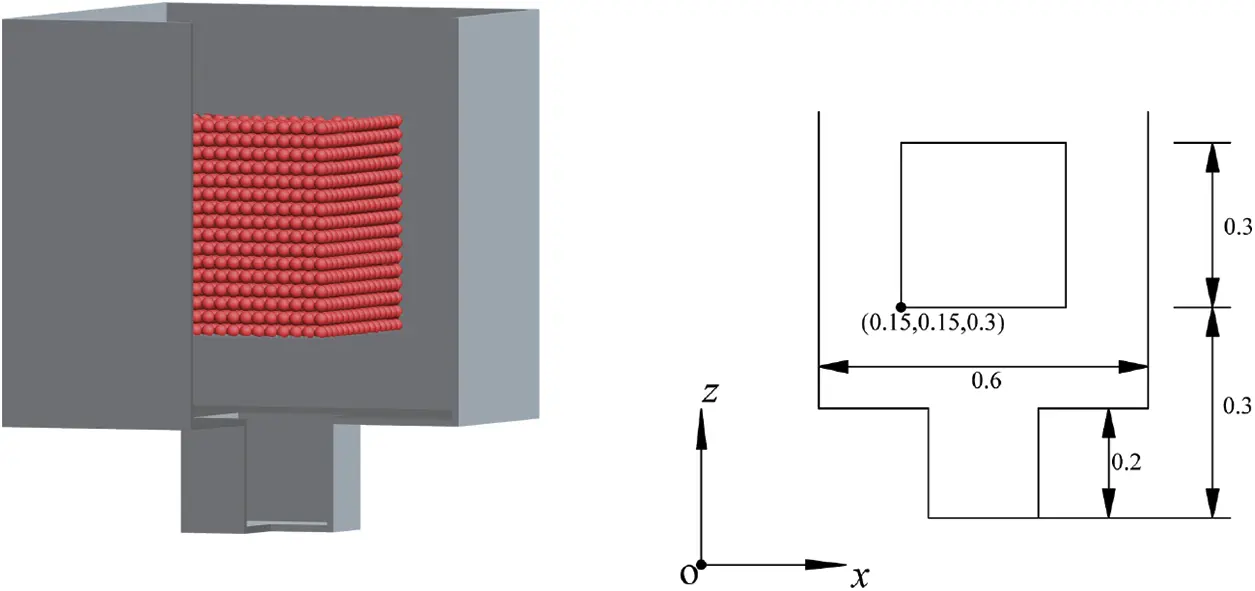Figure 6: Schematic of the problem (Numerical example)

In this section, the consistent time level implementation and preserving the second-order time accuracy for the presented results in this section are as follows for a wide variety of time integrators in the present unified framework. They yield no overshoot in displacement or no overshoot in velocity or no overshoot in both displacement and velocity for a given set of initial conditions:

•   CDM-T: Central Difference Method with traditional tangential displacement evaluation. Note that the central difference method has been proved to be equivalent to the GS4-II U0-based family with (ρmin,ρmax,ρs)=(1,1,0).

•   CDM-C: Central Difference Method with consistent tangential displacement evaluation.

•   V0(1, 1, 0)-C: GS4-II V0-based family with (ρmin,ρmax,ρs)=(1,1,0) and consistent tangential displacement evaluation.

•   U0/V0(0, 1, 0)-C: GS4-II U0-based family or V0-based family with (ρmin,ρmax,ρs)=(0,1,0) and consistent tangential displacement evaluation.

Numerical Setting and Computational Cost

In order to capture particle contacts, the time step size used in DEM type simulations is always required to be very small. This implies that the implicit time integration schemes are not necessary in the general DEM simulations. Therefore, in this work, all the schemes listed are explicit and the time step size is Δt=0.0001 within the total simulation duration from t0=0 to tf=2. As mentioned before, the neighborhood particle-detecting process in any particle-based method is the most expensive step in the simulation. In this paper, the linked list algorithm is used to optimize the neighboring particle searching and decrease the computational time, and parallel computing is also applied to improve the computational performance of our code. Our 3D cases were run on a 1.80 GHz, Intel(R) Core(TM) i7-8565K CPU with 8 GB RAM. Table 1 summarizes the specific timings used for all our 3D examples and shows that our proposed GSSSS family of algorithms does not increase computationally cost in comparison to the traditional algorithms, while our proposed method can obtain better computational accuracy (see Fig. 5).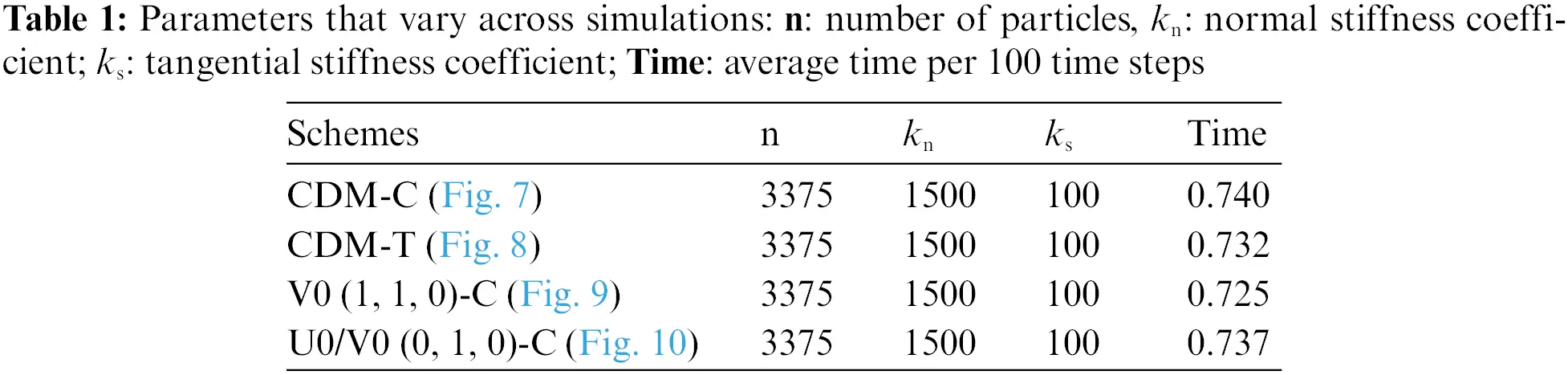Numerical Results and Discussion

Three-dimensional snapshots with the granular velocity filed values at six different times (t=0.20, 0.25, 0.30, 0.40, 0.50 and 1.25) by different schemes are shown in Figs. 7 to 10, respectively. The specifics of particle filling process is observed from 0.2 to 0.5 and the final particle distribution is obtained at time 1.25. The process of particle filling the bottom space corresponds to the evolution of total kinetic energy of the system shown in Fig. 11 (left). As expected, the results of different schemes are very close at each time and the particle distribution shows good physical reliability of the simulation results. In order to better understand the dynamic responses of the granular cube falling process and the computational performance of different schemes, the time history of the total kinetic energy with different schemes are given in Fig. 11 (left). Because the central portion of the granular cube keeps charging into the bottom empty space, the total kinetic energy does not decrease immediately, but a short duration (from 0.17 to 0.23) of energy relaxing instead. After the bottom space is filled with particles, the total kinetic energy decreases sharply though some particles on the top layers still keep bouncing. As the frictional force and damping are considered in the particle contact model, the total kinetic energy converges to zero as time goes.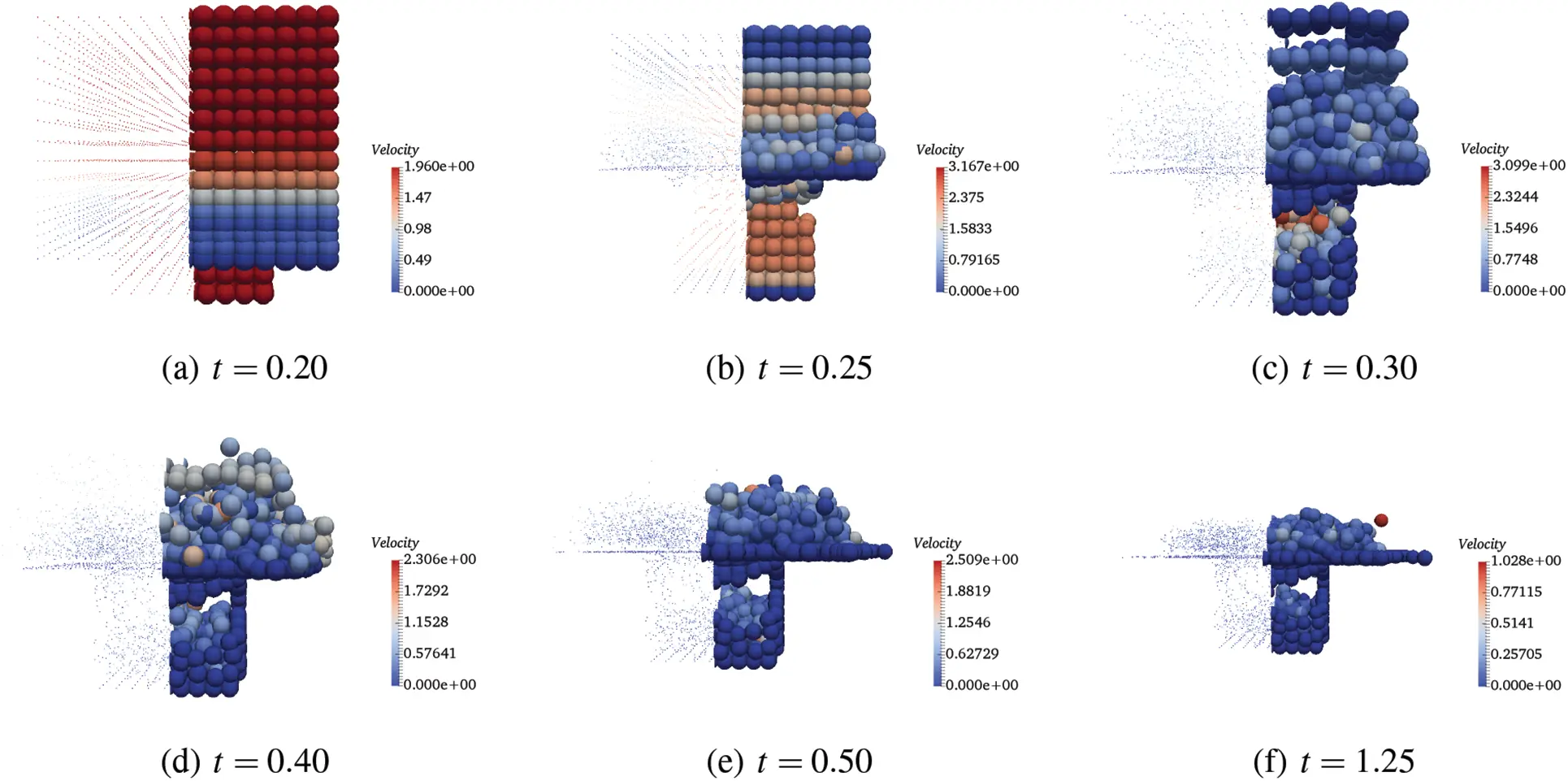Figure 7: Velocity fields at different time by CDM-C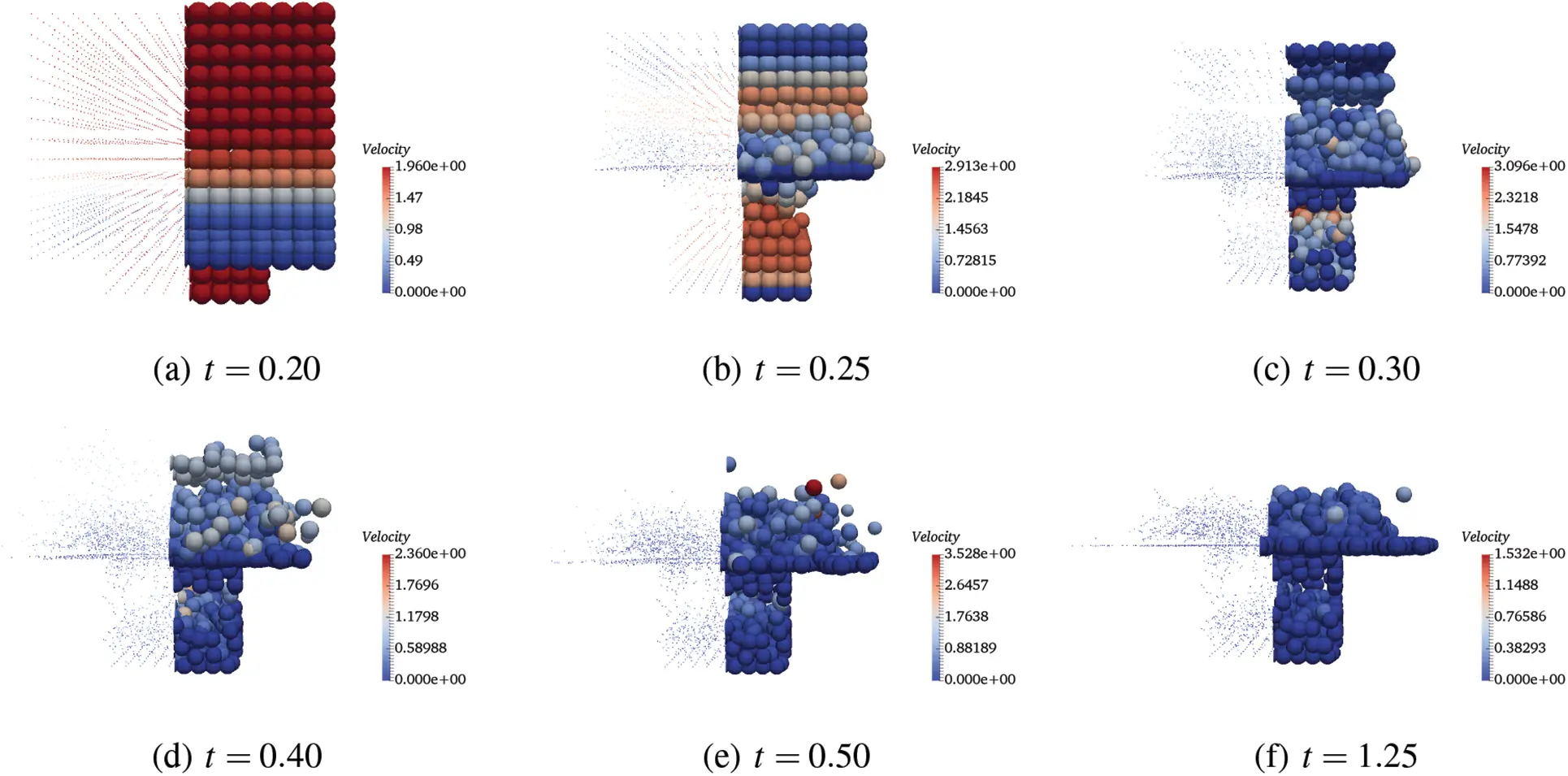Figure 8: Velocity fields at different time by CDM-T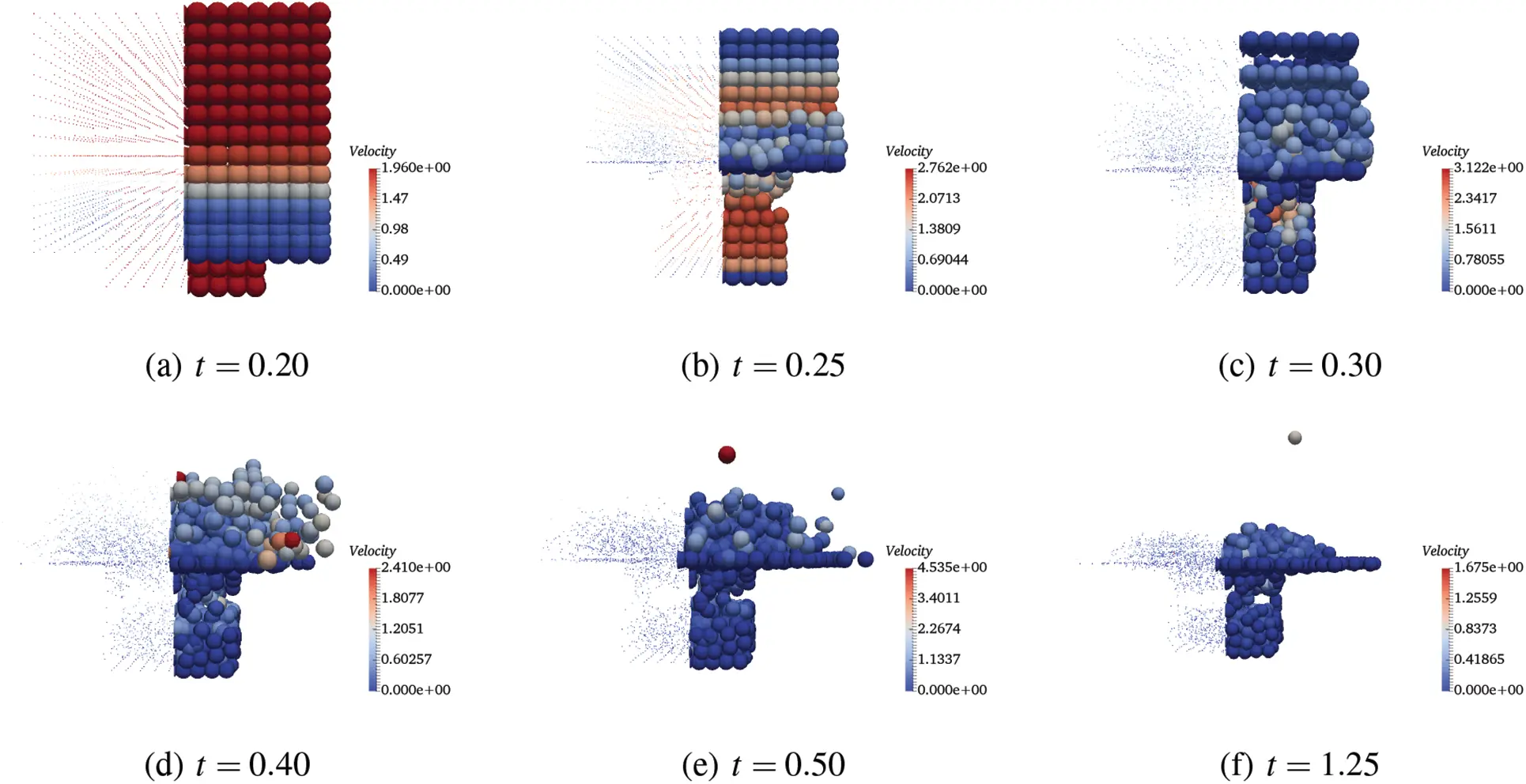Figure 9: Velocity fields at different time by V0(1, 1, 0)-C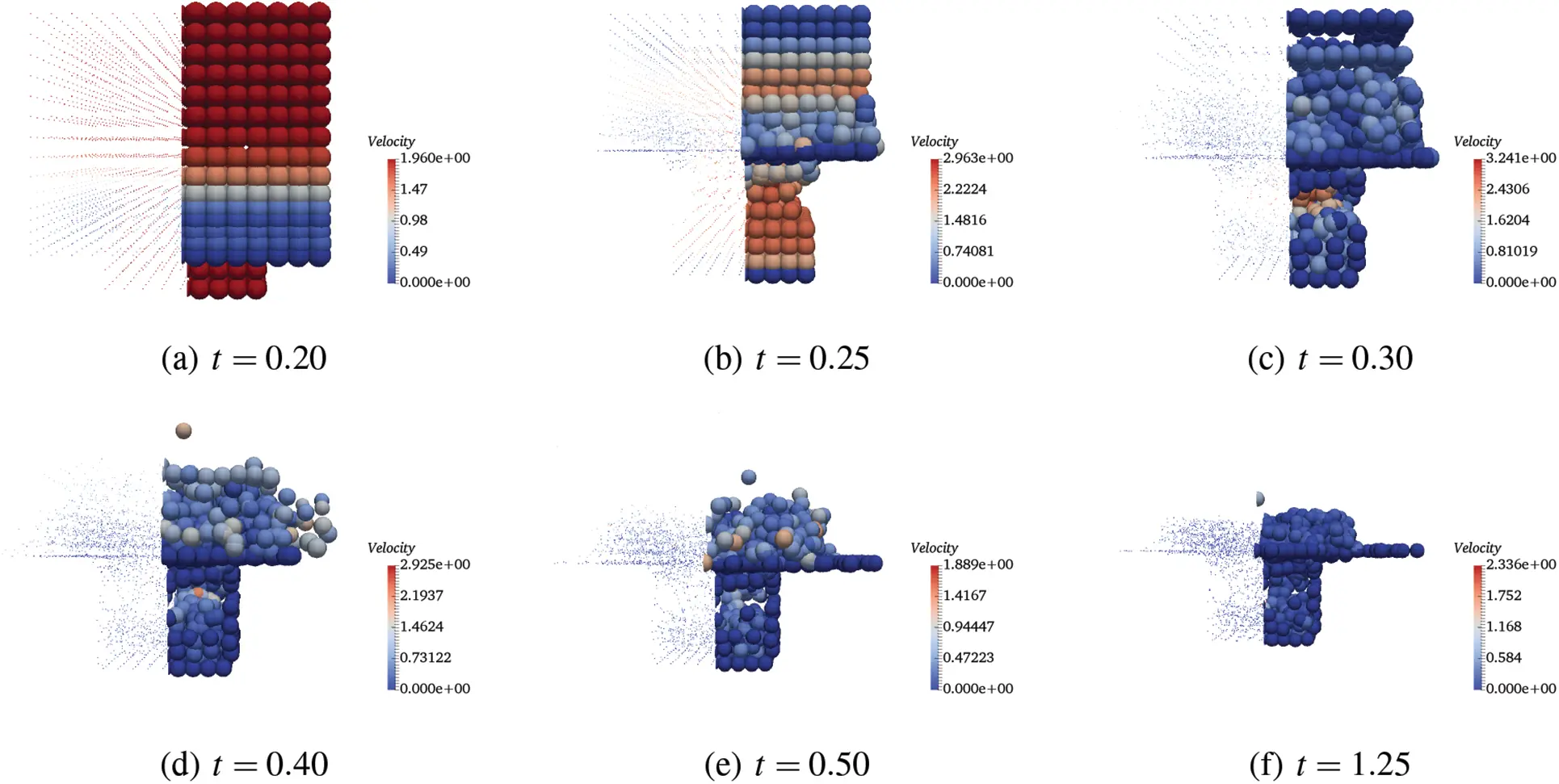Figure 10: Velocity fields at different time by U0/V0(0, 1, 0)-C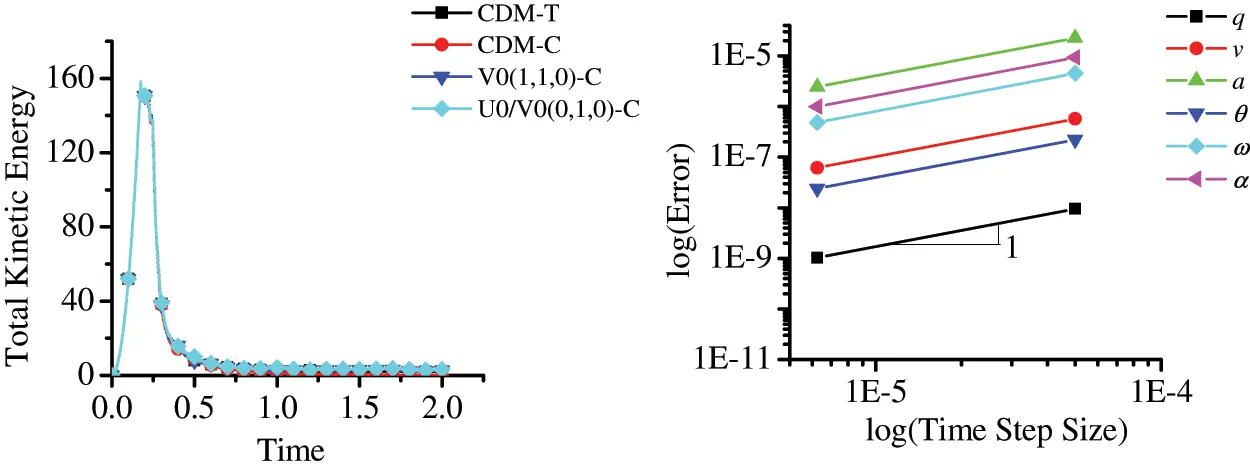Figure 11: Left: total kinetic energy. Right: time accuracy plots of central different method with traditional tangential displacement evaluation

The time accuracy for translational and rotational position, velocity and acceleration of the central difference method with the traditional tangential displacement evaluation shows only first-order time accuracy in Fig. 11 (right). However, alternately, Fig. 12 shows the second-order time accuracy for translational position, velocity and acceleration of different time integration schemes selected within the GS4-II family with the proposed consistent tangential displacement evaluation. All the schemes show the second-order time accuracy in both translational and rotational motion. It is to be noted however, that using central difference method in solving the second-order ODE, it is supposed to yield second-order accuracy in time. According to the time convergence order from different tangential displacement evaluations, it implies that the drawback via the traditional tangential displacement evaluation in DEM type method is not at the same time level as the algorithm and has significant influence on the whole system’s time accuracy. In other words, only when the tangential displacement evaluation is evaluated at a consistent time level corresponding to the selected time integration scheme within the unified framework described, the results are completely reliable and the time integration schemes are implemented correctly and efficiently maintaining second-order time accuracy with improvement in physics.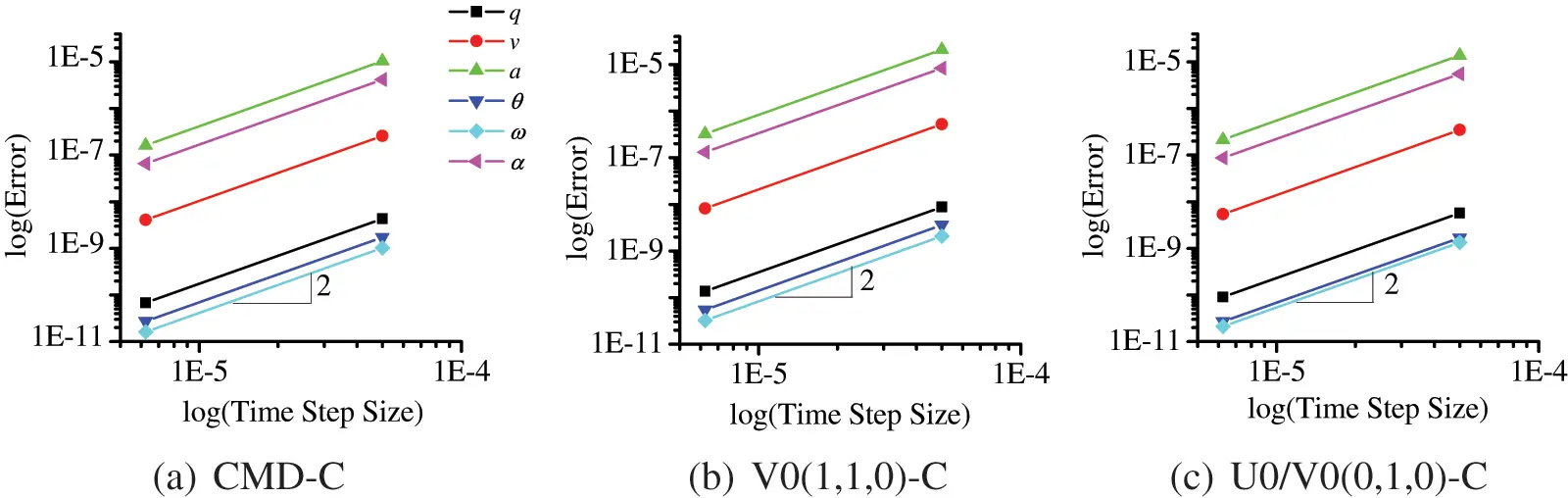Figure 12: Time accuracy plots of different selected schemes within GS4-II family: q, v and a are translational position, velocity and acceleration, respectively. θ, ω and α are angular displacement, velocity and acceleration, respectively

4  Concluding Remarks

In this work, a consistent tangential displacement evaluation approach is proposed under the framework of the unified second-order time integrators GS4-II family for improving discrete element method (DEM) simulations. The newly proposed approach is derived and designed to maintain the simplicity and generalization of the GS4-II family. Comparing it with the traditional tangential displacement evaluation method, the proposed consistent time level implementation approach can be easily implemented and yields the correct algorithmic tangential displacement at the same time level as in the time integrations via using the same parameters (ρmin,ρmax,ρs) as the GS4-II family. The improved time accuracy of both the translational and rotational motions by using the proposed method gives second-order accuracy in time, which is consistent with the time integration schemes; whereas, the traditional second-order time integration method such as the central difference can only obtain first-order accuracy in time via using the traditional tangential displacement evaluation.

Acknowledgement: We appreciate all the constructive comments and suggestions from editors and reviewers.

Funding Statement: The authors received no specific funding for this study.

Conflicts of Interest: The authors declare that they have no conflicts of interest to report regarding the present study.

## References

1. Cundall, P. A., & Strack, O. D. L. (1979). A discrete numerical model for granular assemblies. Geotechnique, 29, 47-65. [Google Scholar] [CrossRef]
2. Luding, S. (1997). Stress distribution in static two-dimensional granular model media in the absence of friction. Physical Review E, 55, 4720-4729. [Google Scholar] [CrossRef]
3. Baxter, J., Tüzün, U., Burnell, J., & Heyes, D. M. (1997). Granular dynamics simulations of two-dimensional heap formation. Physical Review E, 55(3), 3546. [Google Scholar] [CrossRef]
4. Zhou, Y. C., Xu, B. H., Zou, R., Yu, A. B., & Zulli, P. (2003). Stress distribution in a sandpile formed on a deflected base. Advanced Powder Technology, 14(4), 401-410. [Google Scholar] [CrossRef]
5. Fazekas, S., Kertész, J., & Wolf, D. E. (2005). Piling and avalanches of magnetized particles. Physical Review E, 71(6), 061303. [Google Scholar] [CrossRef]
6. Li, Y., Xu, Y., & Thornton, C. (2005). A comparison of discrete element simulations and experiments for ‘sandpiles’ composed of spherical particles. Powder Technology, 160(3), 219-228. [Google Scholar] [CrossRef]
7. Yen, K. Z. Y., & Chaki, T. (1992). A dynamic simulation of particle rearrangement in powder packings with realistic interactions. Journal of Applied Physics, 71(7), 3164-3173. [Google Scholar] [CrossRef]
8. Zhang, Z. P., Liu, L. F., Yuan, Y. D., & Yu, A. B. (2001). A simulation study of the effects of dynamic variables on the packing of spheres. Powder Technology, 116(1), 23-32. [Google Scholar] [CrossRef]
9. Latham, J. P., Lu, Y., & Munjiza, A. (2001). A random method for simulating loose packs of angular particles using tetrahedra. Geotechnique, 51(10), 871-879. [Google Scholar] [CrossRef]
10. Silbert, L. E., Grest, G. S., & Landry, J. W. (2002). Statistics of the contact network in frictional and frictionless granular packings. Physical Review E, 66(6), 061303. [Google Scholar] [CrossRef]
11. Munjiza, A. A. (2004). The combined finite-discrete element method. West Sussex, UK: John Wiley & Sons.
12. An, X. Z., Yang, R. Y., Dong, K. J., Zou, R. P., & Yu, A. B. (2005). Micromechanical simulation and analysis of one-dimensional vibratory sphere acking. Physical Review Letters, 95(20), 205502. [Google Scholar] [CrossRef]
13. Just, S., Toschkoff, G., Funke, A., Djuric, D., & Scharrer, G. (2013). Experimental analysis of tablet properties for discrete element modeling of an active coating process. AAPS PharmSciTech, 14(1), 402-411. [Google Scholar] [CrossRef]
14. Lewis, R. W., Gethin, D. T., Yang, X. S., & Rowe, R. C. (2005). A combined finite-discrete element method for simulating pharmaceutical powder tableting. Numerical Methods in Engineering, 62, 853-869. [Google Scholar] [CrossRef]
15. Buchholtz, V., Pöschel, T., & Tillemans, H. J. (1995). Simulation of rotating drum experiments using non-circular particles. Physica A: Statistical Mechanics and its Applications, 216(3), 199-212. [Google Scholar] [CrossRef]
16. Pöschel, T., & Buchholtz, V. (1995). Complex flow of granular material in a rotating cylinder. Chaos, Solitons and Fractals, 5(10), 1901-1912. [Google Scholar] [CrossRef]
17. Sakai, M., Shibata, K., & Koshizuka, S. (2006). Development of a criticality evaluation method involving the granular flow of the nuclear fuel in a rotating drum. Nuclear Science and Engineering, 154(1), 63-73. [Google Scholar] [CrossRef]
18. Portillo, P. M., Muzzio, F. J., & Ierapetritou, M. G. (2007). Hybrid DEM-compartment modeling approach for granular mixing. AIChE Journal, 53(1), 119-128. [Google Scholar] [CrossRef]
19. Mishra, B. K., & Rajamani, R. K. (1992). The discrete element method for the simulation of ball mills. Applied Mathematical Modelling, 16, 598-604. [Google Scholar] [CrossRef]
20. Inoue, T., & Okaya, K. (2012). Grinding mechanism of centrifugal mills-A simulation study based on the discrete element method. Comminution, 1994, 425. [Google Scholar] [CrossRef]
21. Gudin, D., Kano, J., & Saito, F. (2007). Effect of the friction coefficient in the discrete element method simulation on media motion in a wet bead mill. Advanced Powder Technology, 18(5), 555-565. [Google Scholar] [CrossRef]
22. Sun, X., Sakai, M., & Yamada, Y. (2013). Three-dimensional simulation of a solid-liquid flow by the DEM-SPH method. Journal of Computational Physics, 248, 147-176. [Google Scholar] [CrossRef]
23. Schwartz, S. R., Michel, P., & Richardson, D. C. (2013). Numerically simulating impact disruptions of cohesive glass bead agglomerates using the soft-sphere discrete element method. Icarus, 226(1), 67-76. [Google Scholar] [CrossRef]
24. Guo, Y., Wassgren, C., Hancock, B., Ketterhagen, W., & Curtis, J. (2015). Computational study of granular shear flows of dry flexible fibres using the discrete element method. Journal of Fluid Mechanics, 775, 24-52. [Google Scholar] [CrossRef]
25. Guo, Y., & Curtis, J. S. (2015). Discrete element method simulations for complex granular flows. Annual Review of Fluid Mechanics, 47, 21-46. [Google Scholar] [CrossRef]
26. Munjiza, A., Owen, D., & Bicanic, N. (1995). A combined finite-discrete element method in transient dynamics of fracturing solids. Engineering Computations, 12(2), 145-174. [Google Scholar] [CrossRef]
27. Peters, B. (2013). The extended discrete element method (XDEM) for multi-physics applications. Scholarly Journal of Engineering Research, 2, 1-20. [Google Scholar]
28. Wang, Y., Tamma, K., Maxam, D., Xue, T., & Qin, G. (2021). An overview of high-order implicit algorithms for first-/second-order systems and novel explicit algorithm designs for first-order system representations. Archives of Computational Methods in Engineering, 28(5), 3593-3619. [Google Scholar] [CrossRef]
29. Xue, T., Wang, Y., Aanjaneya, M., Tamma, K. K., & Qin, G. (2021). On a generalized energy conservation/dissipation time finite element method for Hamiltonian mechanics. Computer Methods in Applied Mechanics and Engineering, 373, 113509. [Google Scholar] [CrossRef]
30. Shimada, M., & Tamma, K. K. (2013). Explicit time integrators and designs for first/second order linear transient systems. Encyclopedia of Thermal Stresses, 3, 1524-1530. [Google Scholar] [CrossRef]
31. Atluri, S. N., Han, Z. D., & Shen, S. (2003). Meshless local petrov-galerkin (MLPG) approaches for solving the weakly-singular traction and displacement boundary integral equations. Computer Modeling in Engineering & Sciences, 4(5), 507-518. [Google Scholar] [CrossRef]
32. Han, Z. D., & Atluri, S. (2004). Meshless local petrov-galerkin (MLPG) approaches for solving 3D problems in elasto-statics. Computer Modeling in Engineering & Sciences, 6, 169-188. [Google Scholar] [CrossRef]
33. Long, S., & Atluri, S. N. (2002). A meshless local petrov-galerkin method for solving the bending problem of a thin plate. Computer Modeling in Engineering & Sciences, 3(1), 53-64. [Google Scholar] [CrossRef]
34. Xue, T., Zhang, X., & Tamma, K. K. (2018). A two-field state-based peridynamic theory for thermal contact problems. Journal of Computational Physics, 374, 1180-1195. [Google Scholar] [CrossRef]
35. Xue, T., Zhang, X., & Tamma, K. K. (2019). A non-local dissipative lagrangian modelling for generalized thermoelasticity in solids. Applied Mathematical Modelling, 73, 247-265. [Google Scholar] [CrossRef]
36. Matuttis, H., Chen, J. (2014). Understanding the discrete element method: Simulation of non-spherical particles for granular and multi-body systems, 1st edition. Chichester, UK: John Wiley.
37. Tsuji, Y., Tanaka, T., & Ishida, T. (1992). Lagrangian numerical simulation of plug flow of cohesionless particles in a horizontal pipe. Powder Technology, 71, 239-250. [Google Scholar] [CrossRef]
38. Mindlin, R. D. (1949). Compliance of elastic bodies in contact. Journal of Applied Mechanics, 16, 259-268. [Google Scholar] [CrossRef]
39. Mindlin, R. D., & Deresiewicz, H. (1953). Elastic spheres in contact under varying oblique forces. Journal of Applied Mechanics, 20, 327-344. [Google Scholar] [CrossRef]
40. Har, J., Tamma, K. K. (2012). Advances in computational dynamics of particles, materials and structures. Chichester, UK: John Wiley.
41. Tamma, K. K., Har, J., Zhou, X., Shimada, M., & Hoitink, A. (2011). An overview and recent advances in vector and scalar formalisms: Space/time discretizations in computational dynamics: A unified approach. Archives of Computational Methods in Engineering, 18, 119-283. [Google Scholar] [CrossRef]
42. Shimada, M., Hoitink, A., & Tamma, K. K. (2015). The fundamentals underlying evaluation of acceleration computations for general dynamic applications: Issues and noteworthy perspectives. Computer Methods in Applied Mechanics and Engineering, 104(2), 133-158. [Google Scholar] [CrossRef]
43. Hughes, T. J. R. (2000). The finite element method: Linear static and dynamic finite element analysis. Mineola, New York: Dover Publications.

Xue, T., Wang, Y., Shimada, M., Tae, D., Tamma, K. et al. (2023). A Consistent Time Level Implementation Preserving Second-Order Time Accuracy via a Framework of Unified Time Integrators in the Discrete Element Approach. CMES-Computer Modeling in Engineering & Sciences, 134(3), 1469–1487.This work is licensed under a Creative Commons Attribution 4.0 International License , which permits unrestricted use, distribution, and reproduction in any medium, provided the original work is properly cited.

View

Like

## Related articles

• A. Brasiliano, W.R. Souza, G.N....
• Xue-cong Liu, Qing Zhang, Xiao-zhou...
• A. Rezaei Mojdehi, A. Darvizeh,...
• Chih-Wen Chang
• Chein-Shan Liu